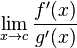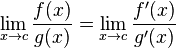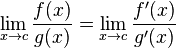• Zill1

#### Zill1

I'm retaking Calculus I right now during the summer. It's been quite a while but I am pretty much breezing through it except for the limit section. I remember the first time around, my prof didn't put ANY emphasis on limits and I haven't really had to do much of anything with limits since then during my math degree. This prof I have now however, seems to love them. Radicalizing, substitution, factoring them; whatever.

My question is this: I noticed that pretty much any limit he's given me I am able to pretty much take the lazy way out and just do L'Hospitals rule for it and it comes out with the same answer whether its an infinite or finite limit. I won't even begin to pretend I know much about limits but is there a specific time in which I CAN'T do L'Hospitals? Obviously if I look at a single limit like (×-1)^2 I'm not going to start using chain rule on it, but what are my restrictions?

As you get further in math, you often go back over old ideas and inspect them more carefully. So limits always have a place in courses later on, and your instructor is enjoying introducing you to this, perhaps at this level, subtle concept. But the topic is a source of many related concepts (for instance multivariable limits) and weird examples.

I'm not sure why you mentioned chain rule. I thought you were talking about l'Ho(s)pital's rule. But if you want to know why the details of the rule are important, try using l'hopital's rule on

$\lim_{x\to0}\frac{\sin(x)}{e^x}.$

The limit should be zero, but using l'Hopital's rule (incorrectly) can give you one.

Obviously things like $\frac{\sin |x|}{|x|}$ as $x \rightarrow 0$ are problems.

$\frac{x + \sin x}{x}$ as $x \rightarrow \infty$ is another famous one.

$\frac{x + \sin x}{x}$ as $x \rightarrow \infty$ is another famous one.
OK, what's the trick here? It seems like the limit meets the conditions of L'hopital's rule, yet it doesn't work. What's going on?

I think you're right lugita15, the limit is one before and after application of l'Ho(s)pital's rule

OK, what's the trick here? It seems like the limit meets the conditions of L'hopital's rule, yet it doesn't work. What's going on?

The limitmust exist in order forto be true. So since limx→∞(1+cos x)/1 doesn't exist, l'Hôpital's rule doesn't apply to the original problem.

http://en.wikipedia.org/wiki/L'Hôpital's_rule

The limitmust exist in order forto be true. So since limx→∞(1+cos x)/1 doesn't exist, l'Hôpital's rule doesn't apply to the original problem.

http://en.wikipedia.org/wiki/L'Hôpital's_rule
OK, I don't think I ever learned this condition.